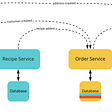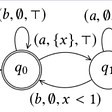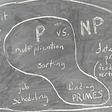# Type Inference by Example, Part 2

Continuing where we left off in part 1, let’s look at a more advanced example that uses local variables and type constructors:

`function range(from, to) {    let numbers = [];    for(let i = from; i <= to; i++) {        numbers[i - from] = i;    }    return numbers;}`

As in the previous installment, we add in fresh type variables:

`function range(from : \$1, to : \$2) : \$3 {    let numbers : \$4 = [];    for(let i : \$5 = from; i <= to; i++) {        numbers[i - from] = i;    }    return numbers;}`

Now, on the very first line, we encounter the empty array:

`let numbers : \$4 = [];`

We know that it should be an Array<… something …>, but what is something? Why, a fresh type variable of course! So for this line, we get the following constraint:

`\$4 == Array<\$6>`

The next line starts the for loop:

`for(let i : \$5 = from; i <= to; i++) {`

Wow, three statements in one line! Assuming that `++ : Int => Int`, this gives us three constraints:

`\$5 == \$1\$5 == \$2\$5 == Int`

The for loop body assigns a value to an index in `numbers`:

`numbers[i - from] = i;`

This tells us two things: Numbers must be an array, and its element type must be the same as the type of `i`.

`\$4 == Array<\$5>`

From the same line, assuming `— : (Int, Int) => Int` we also constrain the types of `i` and `from` to be integers:

`\$5 == Int\$1 == Int`

And finally, from the return statement, we constrain the return type to be the same as the type of `number`:

`\$3 == \$4`

Now that we have generated all the constraints, let’s consider them all together:

`\$4 == Array<\$6>\$5 == \$1\$5 == \$2\$5 == Int\$4 == Array<\$5>\$5 == Int\$1 == Int\$3 == \$4`

Again, unification finds a substitution that solve the above system of equations, but let’s first consider the two constraints involving `Array`. Because `\$4` is on the other side of both equations, we can simplify that to `Array<\$6> == Array<\$5>`. Since both use a type constructor named `Array`, we check the generic arguments against each other by generating the constraint `\$6 == \$5`. And we already have another constraint that `\$5 == Int`. The resulting substitution is thus:

`\$1 := Int\$2 := Int\$3 := Array<Int>\$4 := Array<Int>\$5 := Int\$6 := Int`

Applying that to our syntax tree, we get:

`function range(from : Int, to : Int) : Array<Int> {    let numbers : Array<Int> = [];    for(let i : Int = from; i <= to; i++) {        numbers[i - from] = i;    }    return numbers;}`

And we’re done.

Stay tuned for part 3, where we’re going to look generic function calls.

--

--

--

## More from Joakim Ahnfelt-Rønne

MSc Computer Science, working with functional programming in the industry — github.com/ahnfelt

Love podcasts or audiobooks? Learn on the go with our new app.

## Makers Academy W4 D2: Databasics## Introducing Kudos## Configuring Docker, and configuring a webserver on the Docker container using Ansible## How to Build an NFC-Powered Door Lock with a Raspberry Pi — Switched On Network## We hate queues in shops, but we love them in IT## Understanding Gousto Architecture: The Theory## Characteristics of a Great Scrum Master## Joakim Ahnfelt-Rønne

MSc Computer Science, working with functional programming in the industry — github.com/ahnfelt

## The Arbitrary Substitution Principle## Everything You Need to Know about Angular## Timed Automata and Application## Complexity classes in Algorithms